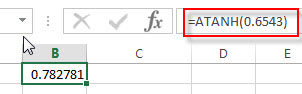# Excel ATANH Function

This post will guide you how to use Excel ATANH function with syntax and examples in Microsoft excel.

### Description

The Excel ATANH function returns the inverse hyperbolic tangent of a given number. So you can use the ATANH function to calculate the inverse hyperbolic tangent for a number. The returned result is a numeric value.

The ATANH function is a build-in function in Microsoft Excel and it is categorized as a Math and Trigonometry Function.

The ATANH function is available in Excel 2016, Excel 2013, Excel 2010, Excel 2007, Excel 2003, Excel XP, Excel 2000, Excel 2011 for Mac.

### Syntax

The syntax of the ATANH function is as below:

`= ATANH (number)`

Where the ATANH function arguments is:

• number -This is a required argument. A real number between 1 and -1, but it should exclude the values of -1 and 1.

Note:

• If the number argument is greater than or equal to 1, the ATANH function will return #NUM! Error. If the number argument is less than or equal to -1, the ATANH function will return #NUM! Error.
• If the supplied number is not a numeric value, the ATANH function will get the #VALUE！ Error。

### Excel ATANH Function Examples

The below examples will show you how to use Excel ATANH Function to return the arctangent of x and y coordinates.

1# get inverse hyperbolic tangent of 0.6543, enter the following formula in Cell B1.

=ATANH(0.6543)The returned result is 0.782781.

### Related Functions

• Excel ATAN function
The Excel ATAN function returns the arctangent of a number. So this function can be used to calculates the arctangent of a supplied number. The returned angle is given in radians in the range –pi/2 to pi/2. The syntax of the ATAN function is as below:= ATAN (number)…
• Excel ATAN2 function
The Excel ATAN2 function returns the arctangent, or inverse tangent, of the specified x- and y-coordinates. And you can use the ATAN2 function to calculate the arctangent of a given x and y coordinates. The syntax of the ATAN2 function is as below:= ATAN2 (x_num, y_num)…
• Excel ACOS function
The Excel ACOS function returns the arccosine value of a number.The ACOS function is a build-in function in Microsoft Excel and it is categorized as a Math and Trigonometry Function.The syntax of the ACOS function is as below:= ACOS(number)…
• Excel ACOSH function
The Excel ACOSH function returns the inverse hyperbolic cosine of a number.The syntax of the ACOSH function is as below:= ACOSH (number)…
• Excel ASIN function
The Excel ASIN function returns the arcsine value of a number.The syntax of the ASIN function is as below:= ASIN (number)…